Symbol
Problem$1$ $2$ $2.$ Find the eigen values and eigen vectors of the $matrix$ $|-6$ $-2$ $-6$ $-12$ $1$ 1 $1$ $3$ $8$ $-12$ $-2$
Algebra
SolutionQanda teacher - NehaMam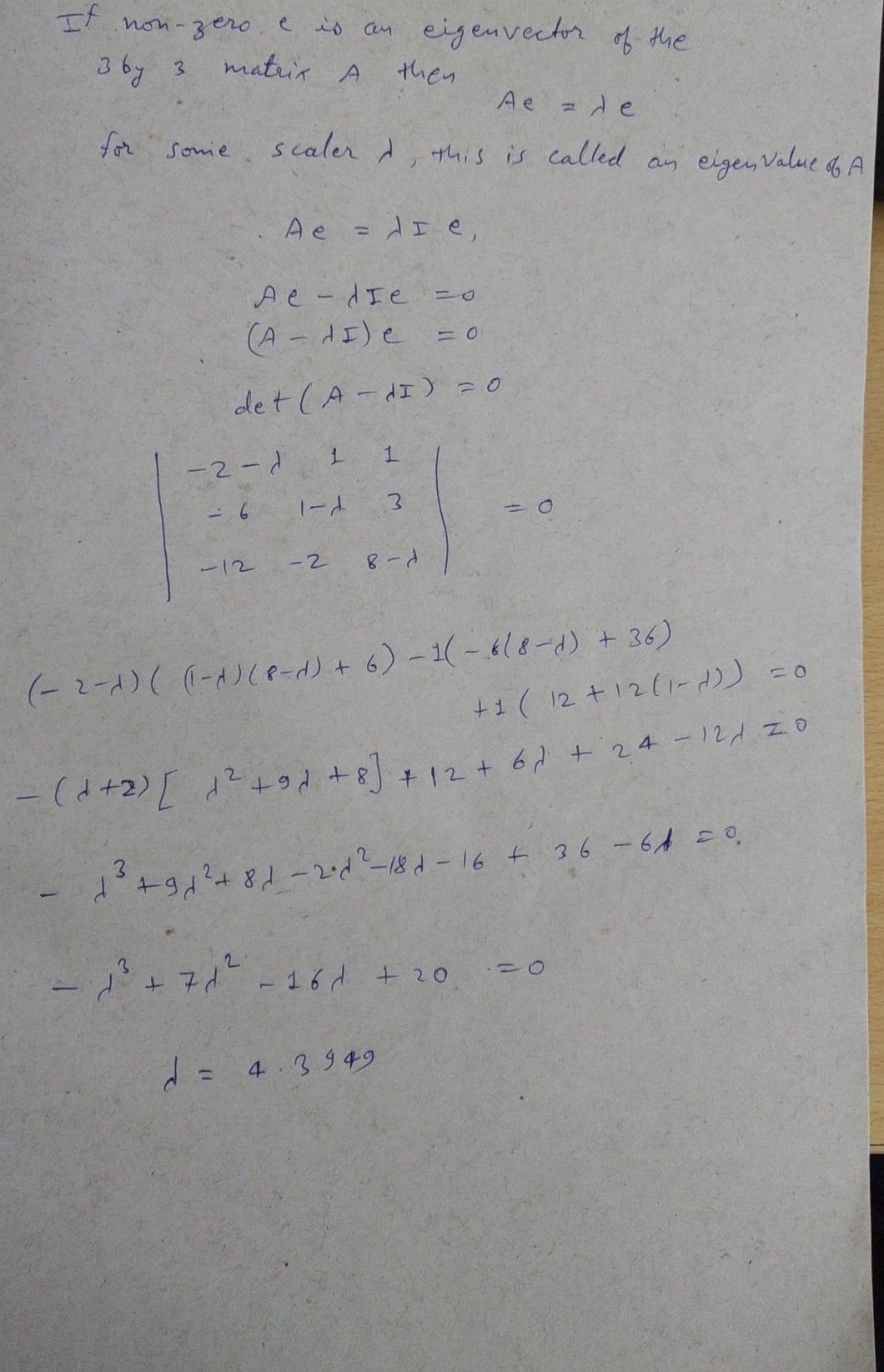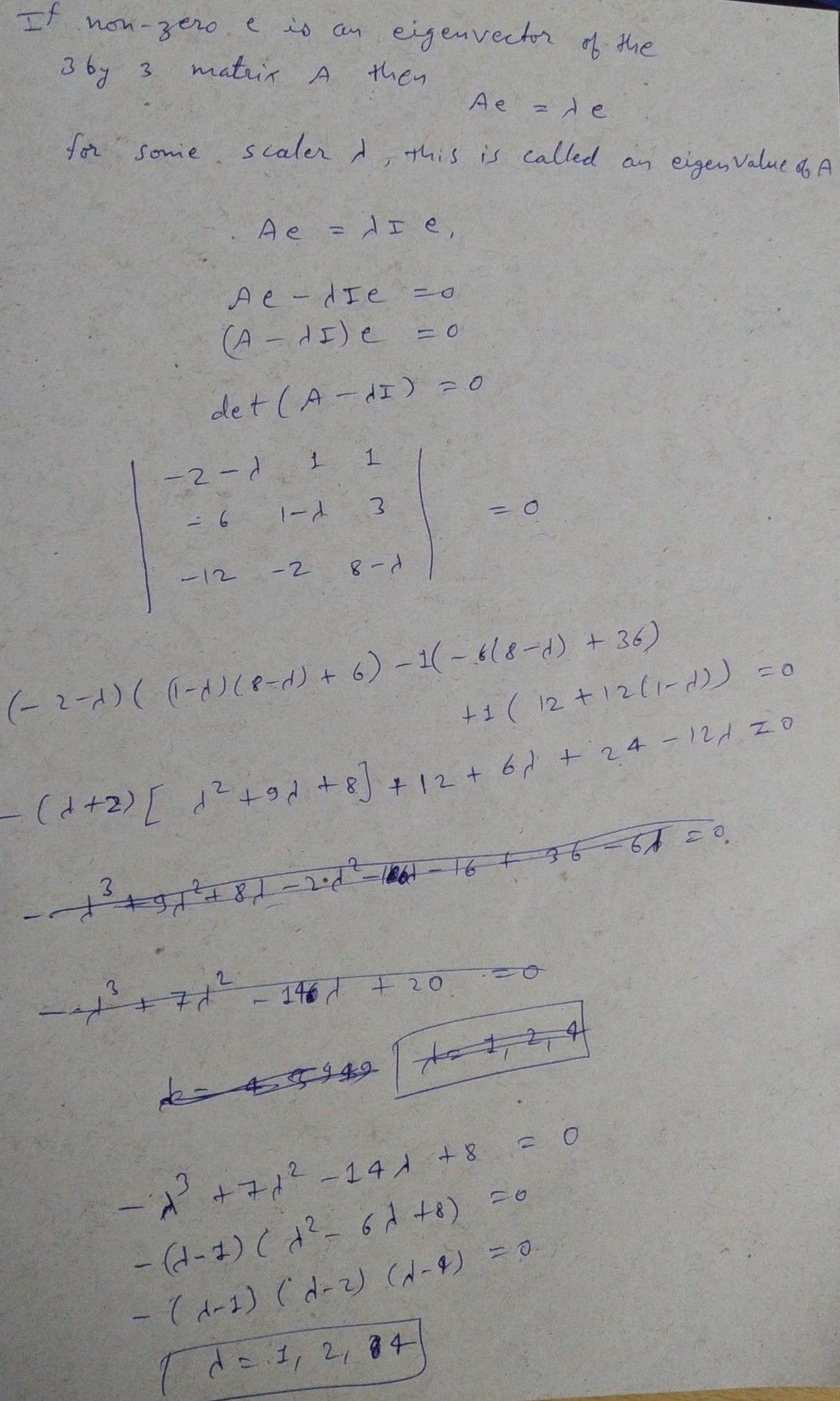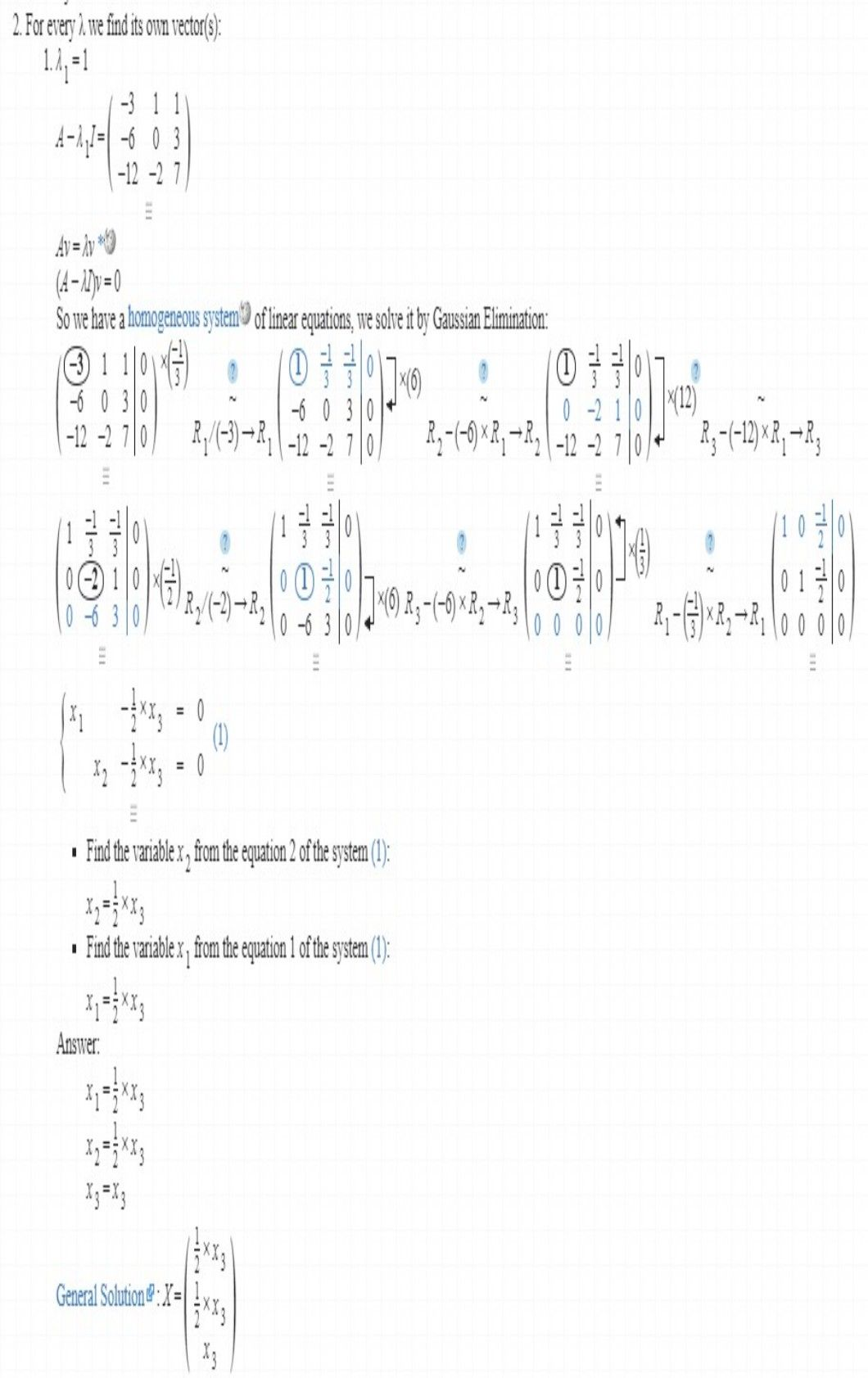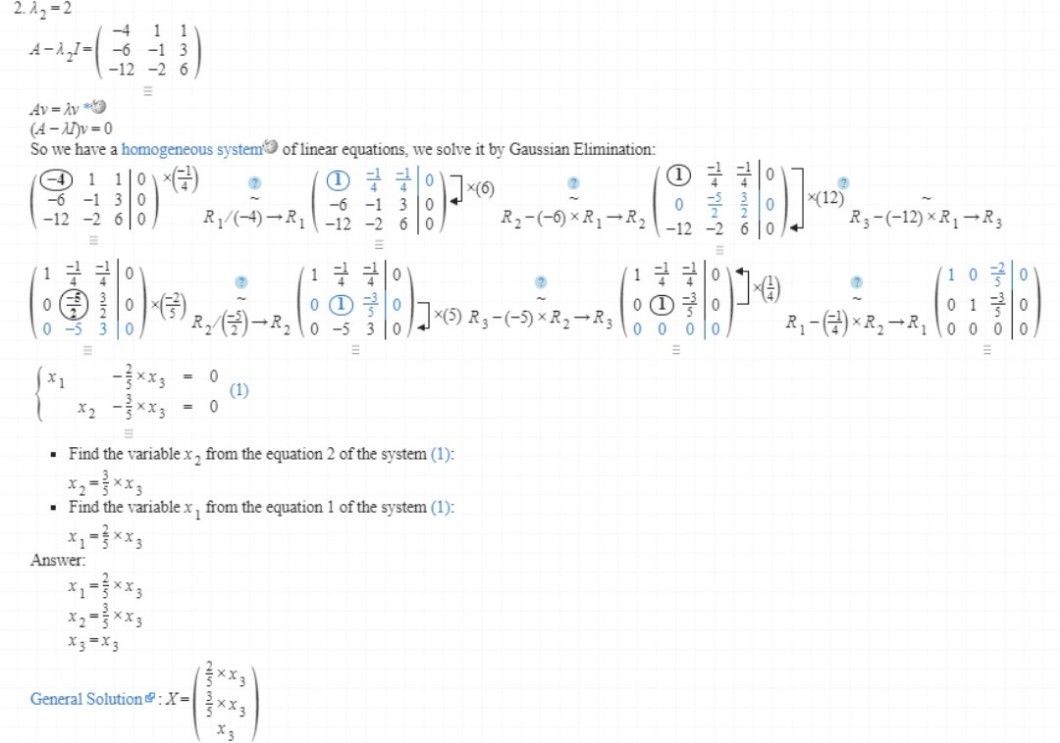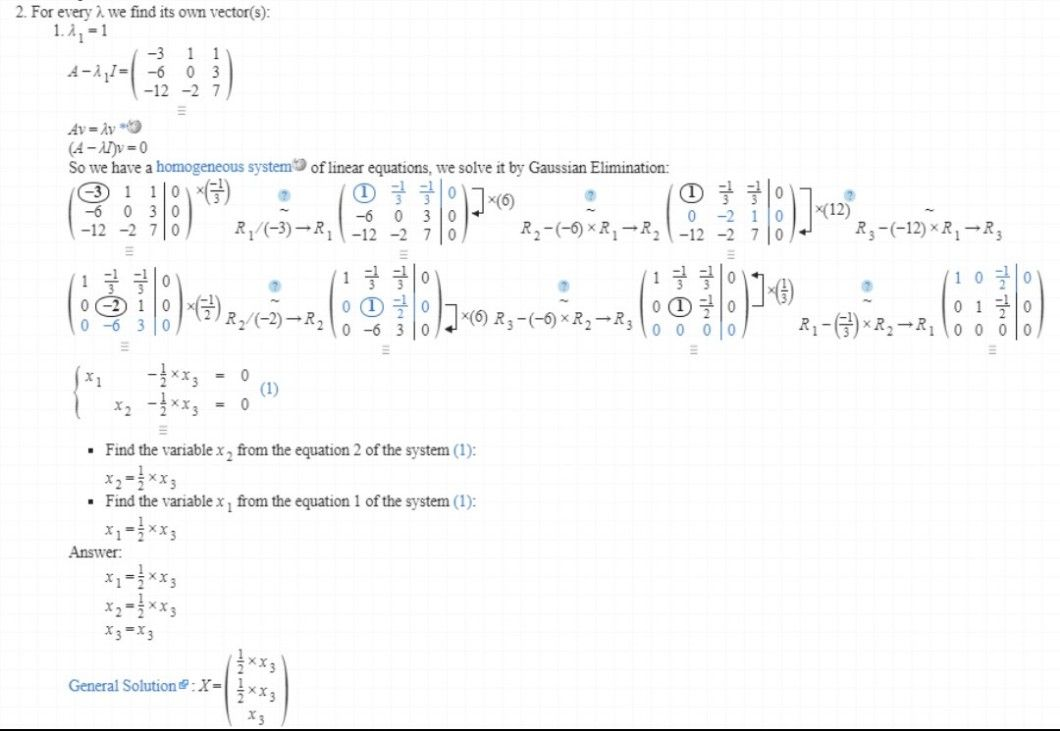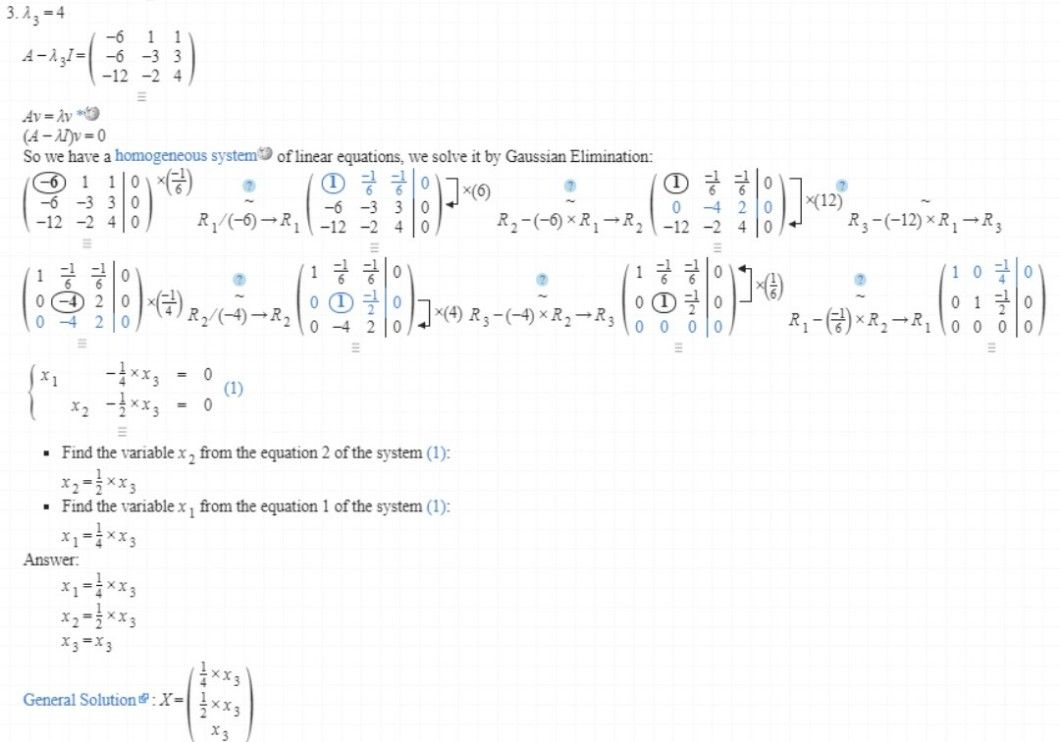If you have doubts in solution feel free to ask if not then please accept the solution and rate it. -thank you